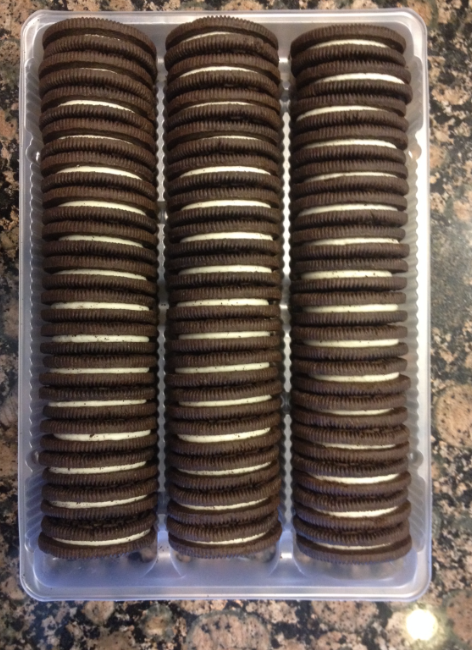Students have been learning to represent numbers as well as skip counting. This week, they tackled the Cookie Monster Problem!! First students were shown the video below and asked what did they wonder (using their math eyes). Students asked: How many cookies came in the package? How many cookies were eaten? How many cookies were left in the package? Next students were asked to estimate how many cookies were left in the package. They were asked to give their estimate, as well as an estimate that was too high and too low. Students placed their yellow stickies on the board to show their estimates. Then students were asked: How many cookies came in the package and how did you count them?Strategies included: -counting each column and adding them (16+16+16=48) -adding 10 rows of 3 (30) plus 6 rows of 3 (18) then 30+18=48 -counting by 2s -16x3 =48 The next day, student watched the video below which summarized the math activity so far: Students were then given the following problem to solve with a partner: Students discussed the math and used chart paper to show how many cookies they thought the Cookie Monster ate!